【Unity3D】Unity3D零基础一步一步教你制作跑酷类游戏

三、正文

Demo源码及对应素材下载：链接: https://pan.baidu.com/s/1smnQFt7 密码: ptcw

1.游戏角色会自动向前跑，玩家可通过左右方向键让其左右移动

2.游戏中存在障碍物，玩家需避开这些障碍物，否则会因为被障碍物阻挡的原因无法前进

3.当游戏角色因为被阻挡而消失在视野中时，视为失败

4.当游戏角色因为被阻挡而处于偏后方时，会提高移动速度直到回到原本所处的屏幕位置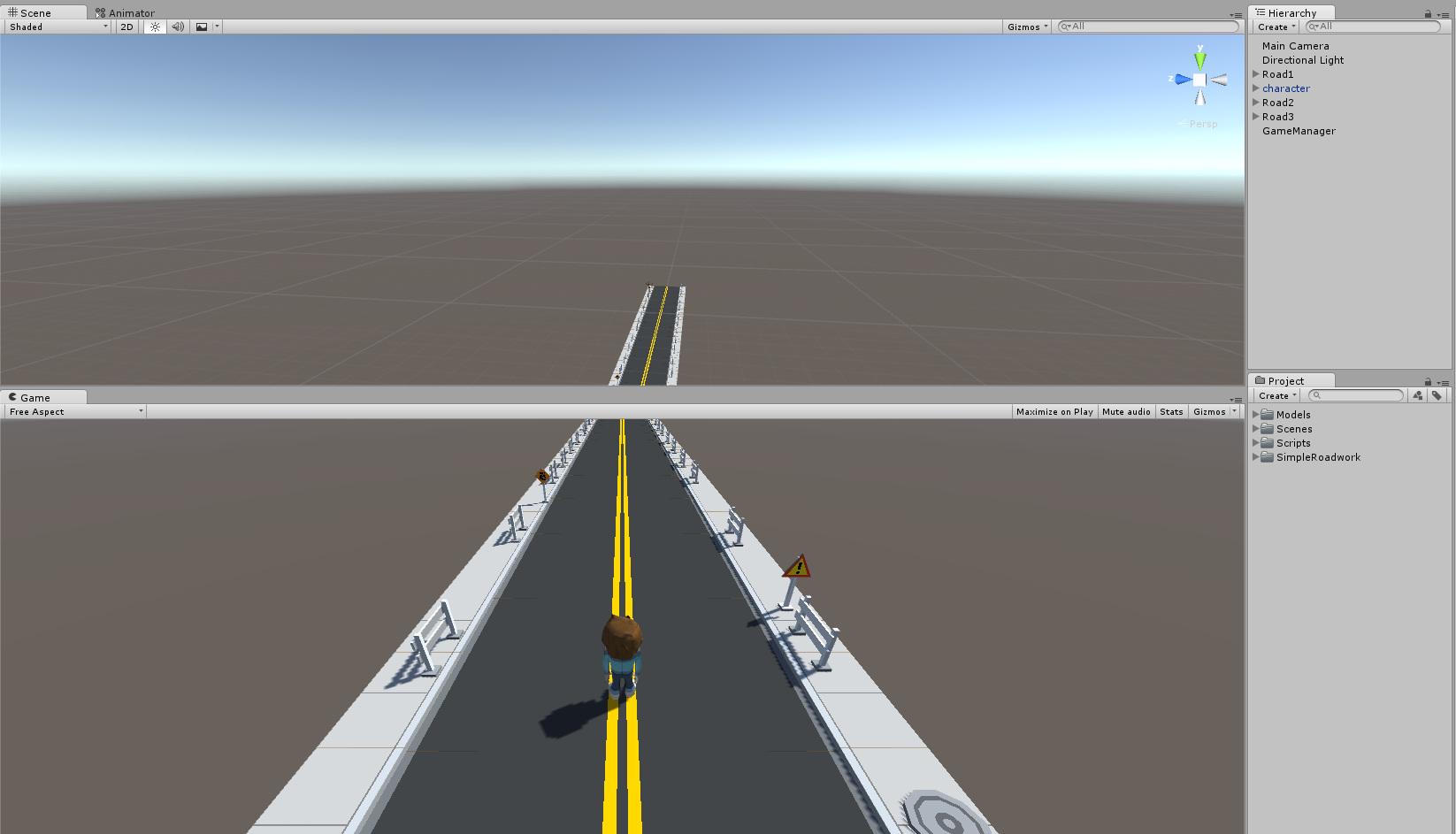// 前进移动速度
float moveVSpeed;
// 水平移动速度
public float moveHSpeed = 5.0f;
// 最大速度
public float maxVSpeed = 10.0f;
// 最小速度
public float minVSpeed = 5.0f;



moveVSpeed = minVSpeed;

        float h = Input.GetAxis("Horizontal");
Vector3 vSpeed = new Vector3(this.transform.forward.x, this.transform.forward.y, this.transform.forward.z) * moveVSpeed ;
Vector3 hSpeed = new Vector3(this.transform.right.x, this.transform.right.y, this.transform.right.z) * moveHSpeed * h;
Vector3 jumpSpeed = new Vector3(this.transform.up.x, this.transform.up.y, this.transform.up.z) * jumpHeight * m_jumpState;
this.transform.position += (vSpeed + hSpeed + jumpSpeed) * Time.deltaTime;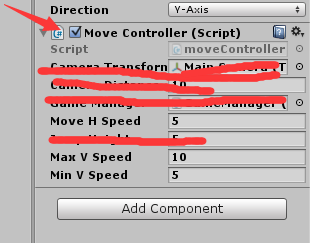// 摄像机位置
public Transform cameraTransform;


// 设置摄像机移动速度
Vector3 vCameraSpeed = new Vector3(this.transform.forward.x, this.transform.forward.y, this.transform.forward.z) * minVSpeed;
// 让摄像机跑起来
cameraTransform.position += (vCameraSpeed) * Time.deltaTime;

1.摄像机没有左右移动
2.摄像机的速度恒定为minVSpeed，也就是我们所定义的人物的最小移动速度（当然这个时候人物也一直是以这个速度在移动）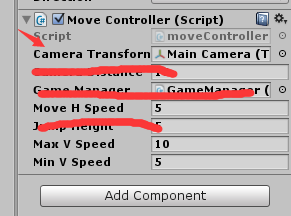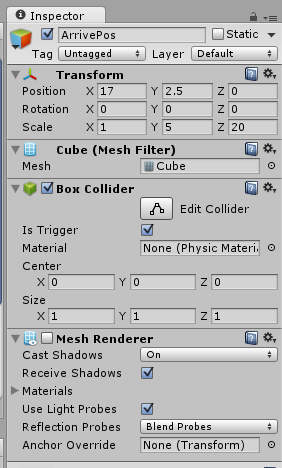// 生成障碍物点列表
public List<Transform> bornPosList = new List<Transform>();
// 道路列表
public List<Transform> roadList = new List<Transform>();
// 抵达点列表
public List<Transform> arrivePosList = new List<Transform>();
// 障碍物列表
public List<GameObject> objPrefabList = new List<GameObject>();
// 目前的障碍物
Dictionary<string, List<GameObject>> objDict = new Dictionary<string, List<GameObject>>();
// 道路间隔距离



    // 切出新的道路
{
int index = arrivePosList.IndexOf(arrivePos);
if(index >= 0)
{
int lastIndex = index - 1;
if (lastIndex < 0)
// 移动道路

}
else
{
Debug.LogError("arrivePos index is error");
return;
}
}

{

// 清空已有障碍物
{
Destroy(obj);
}

// 添加障碍物
foreach(Transform pos in bornPosList[index])
{
GameObject prefab = objPrefabList[Random.Range(0, objPrefabList.Count)];
Vector3 eulerAngle = new Vector3(0, Random.Range(0, 360), 0);
GameObject obj = Instantiate(prefab, pos.position, Quaternion.EulerAngles(eulerAngle)) as GameObject;
obj.tag = "Obstacle";
}
}



    void Start () {
{
List<GameObject> objList = new List<GameObject>();
}
}



// 游戏管理器
public GameManager gameManager;

    void OnTriggerEnter(Collider other)
{
// 如果是抵达点
if (other.name.Equals("ArrivePos"))
{
}
// 如果是透明墙
else if (other.tag.Equals("AlphaWall"))
{
// 没啥事情
}
// 如果是障碍物
else if(other.tag.Equals("Obstacle"))
{

}
}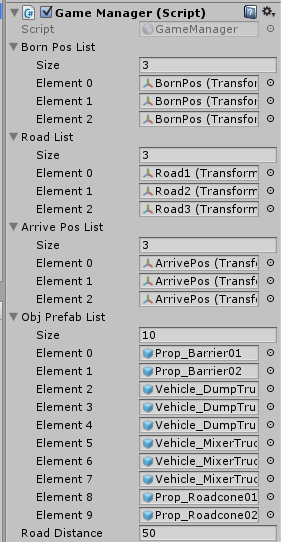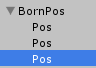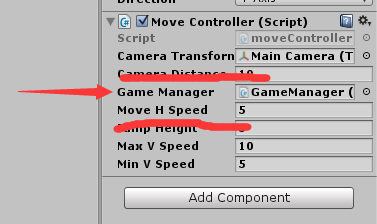public bool isEnd = false;


// 摄像机距离人物的距离


// 当人物与摄像机距离小于cameraDistance时 让其加速
{
moveVSpeed += 0.1f;
if (moveVSpeed > maxVSpeed)
{
moveVSpeed = maxVSpeed;
}
}
// 超过时 让摄像机赶上
else if(this.transform.position.x - cameraTransform.position.x > cameraDistance)
{
moveVSpeed = minVSpeed;
cameraTransform.position = new Vector3(this.transform.position.x - cameraDistance, cameraTransform.position.y, cameraTransform.position.z);
}
// 摄像机超过人物
if(cameraTransform.position.x - this.transform.position.x > 0.0001f)
{
Debug.Log("你输啦！！！！！！！！！！");
gameManager.isEnd = true;
}



void OnGUI()
{
if (gameManager.isEnd)
{
GUIStyle style = new GUIStyle();

style.alignment = TextAnchor.MiddleCenter;
style.fontSize = 40;
style.normal.textColor = Color.red;
GUI.Label(new Rect(Screen.width / 2 - 100, Screen.height / 2 - 50, 200, 100), "你输了~", style);

}
}